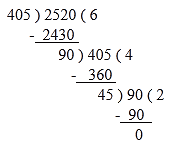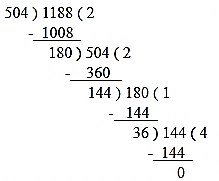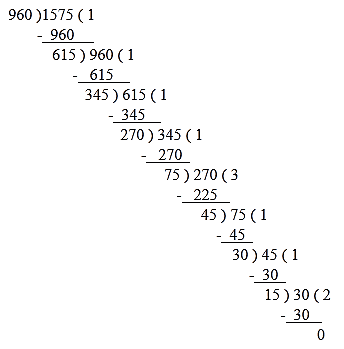RS Aggarwal Class 10 Solutions Chapter 1 Real Numbers

RS Aggarwal Class 10 Chapter 1 Real Numbers Solutions Free PDF

A number which is a continuous quantity that represents a distance along a line and can be used for a variety of different calculations such as velocity, mass and energy. Real numbers can be of either positive numbers, negative numbers, decimals, fractions or even pi. There are quite a few applications of Real numbers such as Physics, Computation, Logic, Computation and Set Theory.

Real numbers include the following types of numbers:

1. Whole Numbers
2. Irrational Numbers
3. Rational Numbers

We have provided step by step solutions of all the exercise questions in the downloadable pdf format. Check out the RS Aggarwal Class 10 Solutions Chapter 1 Real Numbers are available below:

Q.1: What do you mean by Euclid’s division lemma?

Sol:

Euclid’s division algorithm states that for any two positive integers a and b, there exist unique integers Q. and r, such that a = bQ + r, where Q ≠ 0 and r < b.

Q.2: A number when divided by 61 gives 27 as Quotient and 32 as remainder. Find the number.

Sol:

We know, Dividend = Divisor x Quotient + Remainder

Given: Divisor = 61, Quotient = 27, Remainder = 32

Let the Dividend be x.

Therefore, x = 61 x 27 + 32 = 1679

Hence, the required number is 1679.

Q.3: By what number should 1365 be divided to get 31 as Quotient and 32 as remainder?

Sol:

Given: Dividend = 1365, Quotient = 31, Remainder = 32

Let the divisor be x.

Dividend = Divisor x Quotient + Remainder

1365 = 31x + 32

$\Rightarrow$ 1365 – 32 = 31x

$\Rightarrow$ 1333 = 31x

$\Rightarrow$ x = $\frac{ 1333 }{ 31 }$ = 43

Hence, 1365 should be divided by 43 to get 31 as Quotient and 32 as remainder.

Q.4: Using Euclid’s division algorithm, find the HCF of:

(i) 405 and 2520 (ii) 504 and 1188 (iii) 960 and 1575

Sol:

(i)On applying Euclid’s algorithm, i.e. dividing 2520 by 405, we get:

Quotient = 6, Remainder = 90

Therefore, 2520 = 405 x 6 + 90

Again on applying Euclid’s algorithm, i.e. dividing 405 by 90, we get:

Quotient = 4, Remainder = 45

Therefore, 405 = 90 x 4 + 45

Again on applying Euclid’s algorithm, i.e. dividing 90 by 45, we get:

Therefore, 90 = 45 x 2 + 0

Hence, the HCF of 2520 and 405 is 45.

(ii)On applying Euclid’s algorithm, i.e. dividing 1188 by 504, we get:

Quotient = 2, Remainder = 180

Therefore, 1188 = 504.2 +180

Again on applying Euclid’s algorithm, i.e. dividing 504 by 180, we get:

Quotient = 2, Remainder = 144

Therefore, 504 = 180x 2 + 144

Again on applying Euclid’s algorithm, i.e. dividing 180 by 144, we get:

Quotient = 1, Remainder = 36

Therefore, 180 = 144 x 1 + 36

Again on applying Euclid’s algorithm, i.e. dividing 144 by 36, we get:

Therefore, 144 = 36 x 4 + 0

Hence, the HCF of 1188 and 504 is 36.

(iii)On applying Euclid’s algorithm, i.e. dividing 1575 by 960, we get:

Quotient = 1, Remainder = 615

Therefore, 1575 = 960 x 1 + 615

Again on applying Euclid’s algorithm, i.e. dividing 960 by 615, we get:

Quotient = 1, Remainder = 345

Therefore, 960 = 615 x 1 + 345

Again on applying Euclid’s algorithm, i.e. dividing 615 by 345, we get:

Quotient = 1, Remainder = 270

Therefore, 615 = 345 x 1 + 270

Again on applying Euclid’s algorithm, i.e. dividing 345 by 270, we get:

Quotient = 1, Remainder = 75

Therefore, 345 = 270 x 1 + 75

Again on applying Euclid’s algorithm, i.e. dividing 270 by 75, we get:

Quotient = 3, Remainder = 45

Therefore, 270 = 75 x 3 + 45

Again on applying Euclid’s algorithm, i.e. dividing 75 by 45, we get:

Quotient = 1, Remainder = 30

Therefore, 75 = 45 x 1 + 30

Again on applying Euclid’s algorithm, i.e. dividing 45 by 30, we get:

Quotient = 1, Remainder = 15

Therefore, 45 = 30 x 1 + 15

Again on applying Euclid’s algorithm, i.e. dividing 30 by 15, we get:

Quotient = 2, Remainder = 0

Therefore, 30 = 15 x 2 + 0

Hence, the H.C.F of 960 and 1575 is 15.

Q.5: Show that every positive integer is either even or odd.

Sol:

Let us assume that there exists a smallest positive integer that is neither odd nor even, say n. Since n is the least positive integer which is neither even nor odd, n – 1 must be either odd or even.

Case 1: If n – 1 is even, n – 1 = 2k for some k.

But this implies n = 2k + 1

This implies n is odd.

Case 2: If n – 1 is odd, n – 1 = 2k + 1 for some k.

But this implies n = 2k + 2 = 2(k + 1)

This implies n is even.

In both ways, we have a contradiction.

Thus, every positive integer is either even or odd.

Q.6: Show that any positive odd integer is of the form (6m + 1) or (6m + 3) or (6m + 5), where m is some integer.

Sol:

Let n be any arbitrary positive odd integer.

On dividing n by 6, let m be the Quotient and r be the remainder. So, by Euclid’s division lemma, we have

n = 6m + r, where m ≠ 0 and  r < 6.

As m ≠ 0 and  r < 6 and r is an integer, r can take values 0, 1, 2, 3, 4, 5.

$\Rightarrow$n= 6m or n = 6m + 1 or n = 6m + 2 or n = 6m + 3 or n = 6m + 4 or n = 6m + 5

$\Rightarrow$n = 6m + 1 or n = 6m + 3 or n = 6m + 5

Thus, any positive odd integer is of the form (6m + 1) or (6m + 3) or (6m + 5), where m is some integer.

Q.7: Show that any positive odd integer is of the form ( 4m + 1 ) or ( 4m + 3),, where m is some integer.

Sol:

Let n be any arbitrary positive odd integer.

On dividing n by 4, let m be the Quotient and r be the remainder. So, by Euclid’s division lemma, we have

n = 4m + r, where m ≠ 0 and  r < 4.

As m ≠ 0 and  r < 4 and r is an integer, r can take values 0, 1, 2, 3.

$\Rightarrow$ n = 4m or n = 4m + 1 or n = 4m + 2 or n = 4m + 3

$\Rightarrow$n = 4m + 1 or n = 4m + 3

Thus, any positive odd integer is of the form (4m + 1) or (4m + 3), where m is some integer.

Q.8: Using prime factorization, find the HCF and LCF of:

(i) 36, 84 (ii) 23, 31 (iii) 96, 404 (iv) 144, 198 (v) 396, 1080

(vi) 1152, 1664

In each case, verify that:

HCF x LCM = product of given numbers.

Sol:

(i) 36, 84

Prime factorisation:

36 = 22 x 32

84 = 22 x 3 x 7

HCF = product of smallest power of each common prime factor in the numbers = 22 x 3 = 12

LCM = product of greatest power of each prime factor involved in the numbers = 22 x 32 x 7 = 252

(ii) 23, 31

Prime factorization:

23 = 23

31 = 31

HCF = product of smallest power of each common prime factor in the numbers = 1

LCM = product of greatest power of each prime factor involved in the numbers = 23 x 31 = 713

(iii) 96, 404

Prime factorization:

96 = 25 x 3

404 = 22 x 101

HCF = product of smallest power of each common prime factor in the numbers = 22 = 4

LCM = product of greatest power of each prime factor involved in the numbers = 25 x 3 x 101 = 9696

(iv) 144, 198

Prime factorization:

144 = 24 x 32

198 = 2 x 32 x 11

HCF = product of smallest power of each common prime factor in the numbers = 2 x 32 = 18

LCM = product of greatest power of each prime factor involved in the numbers = 24 x 32 x 11 = 1584

(v) 396, 1080

Prime factorization:

396 = 22 x 32 x 11

1080 = 23 x 33 x 5

HCF = product of smallest power of each common prime factor in the numbers = 22 x 32 = 36

LCM = product of greatest power of each prime factor involved in the numbers = 23 x 33 x 5 x 11 = 11880

(vi) 1152, 1664

Prime factorization:

1152 = 27 x 32

1664 = 27 x 13

HCF = product of smallest power of each common prime factor involved in the numbers = 27 = 128

LCM = product of greatest power of each prime factor involved in the numbers = 27 x 32 x 13 = 14976

Q.9: Using prime factorization, find the HCF and LCF of:

(i) 8, 9, 25 (ii) 12, 15, 21 (iii) 17, 23, 29 (iv) 24, 36, 40

(iv) 24, 36, 40 (v) 30, 72, 432 (vi) 21, 28, 36, 45

Sol:

(i) 8 = 2 x 2 x 2 = 23

9 = 3 x 3 = 32

25 = 5 x 5 = 52

HCF = Product of smallest power of each common prime factor in the numbers = 1

LCM = Product of the greatest power of each prime factor involved in the numbers = 23 x 32 x 52 = 1800

(ii) 12 = 2 x 2 x 3 = 22 x 3

15 = 3 x 5

21 = 3 x 7

HCF = Product of smallest power of each common prime factor in the numbers = 3

LCM = Product of the greatest power of each prime factor involved in the numbers = 22 x 3 x 5 x 7 = 420

(iii) 17 = 17

23 = 23

29 = 29

HCF = Product of smallest power of each common prime factor in the numbers = 1

LCM = Product of the greatest power of each prime factor involved in the numbers = 17 x 23 x 29 = 11339

(iv) 24 = 2 x 2 x 2 x 3 = 23 x 3

36 = 2 x 2 x 3 x 3 = 22 x 32

40= 2 x 2 x 2 x 5 = 23 x 5

Therefore, HCF = Product of smallest power of each common prime factor in the numbers = 22 = 4

Therefore, LCM = Product of the greatest power of each prime factor involved in the numbers = 23 x 32 x 5 = 360

(v) 30 = 2 x 3 x 5

72=2x2x2x3x3=23x32

432.2x2x2x2x3x3x3=24 x 33

Therefore, HCF = Product of smallest power of each common prime factor in the numbers = 2 x 3 = 6

Therefore, LCM = Product of the greatest power of each prime factor involved in the numbers = 24 x 33 x 5 = 2160

(vi) 21 = 3 x 7

28 = 28 = 2 x 2 x 7 = 22 x 7

36 = 2 x 2 x 3 x 3 = 22 x 32

45 = 5 x 3 x 3 = 5 x 32

Therefore, HCF = Product of smallest power of each common prime factor in the numbers = 1

Therefore, LCM = Product of the greatest power of each prime factor involved in the numbers = 2x 32 x 5 x 7 = 1260

Q.10: The HCF of two numbers is 23 and their LCM is 1449. If one of the numbers is 161, find the other.

Sol:

Let, the two numbers be a and b.

Let the value of a be 161.

Given: HCF = 23 and LCM = 1449

we know, a x b = HCF x LCM

$\Rightarrow$ 161 x b = 23 x 1449

$\Rightarrow$ Therefore, b = $\frac{23X1449}{161}$ = $\frac{33327}{161}$== 207

Hence, the other number b is 207.

RS Aggarwal Class 10 Solutions Chapter 1 – Real Numbers

The RS Aggarwal Maths Solutions for Class 10 will help you score high marks in your mathematics exam as we focus on providing solutions which will simplify the complex fundamentals of the subject. These solutions are prepared by our highly skilled subject experts strictly following the latest CBSE Class 10 Maths syllabus. From the exam point of view, practicing from RS Aggarwal Maths textbooks will help students boosting their knowledge and academic performance. It will help them enhance their problem-solving skills.

Practise This Question

Consider a point A on the circle of radius 7π cm as shown in the figure. A ball on point A moves along the circumference until it reaches a point B. The tangent at B is parallel to the tangent at A. What is the distance traveled by the ball? (Consider the ball to be a point object)Note: The point B in the diagram may not represent its actual position.